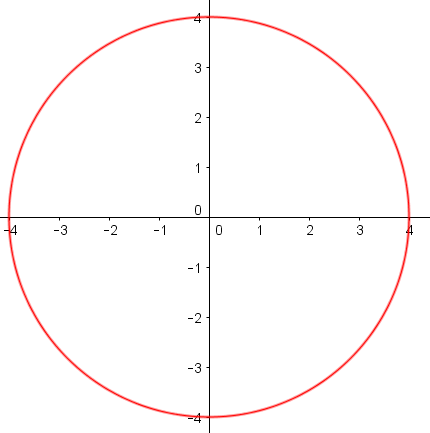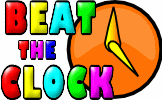# Circle Equations

## Recognise and use the equation of a circle with centre at the origin and the equation of a tangent to a circle.

##### Level 1Level 2Exam-StyleDescriptionHelpMore Graphs

This is level 1: equations of circles.

 1)Which of the following is the equation of the circle above?a) $$x^2 + y^2 = 16$$b) $$x^2 + y^2 = 4$$c) $$x^2 + y^2 = 8$$2) The equation of a circle is $$x^2 + y^2 = 16$$. What is the radius of the circle?3) The equation of a circle is $$x^2 + y^2 = 20.25$$. What is the radius of the circle?4) Which of the following is the equation of a circle with centre at the origin and a radius of 14 units?a) $$x^2 + y^2 = 196$$b) $$x^2 + y^2 = 14$$c) $$x^2 + y^2 = 28$$5) The equation of a circle is $$5x^2 + 5y^2 = 80$$. What is the radius of the circle?6) The equation of a circle is $$7x^2 + 7y^2 = 252$$. What is the radius of the circle?7) Which of the following is the equation of a circle with centre at the origin and a radius of 9 units?a) $$2x^2 + 2y^2 = 81$$b) $$x^2 + y^2 = 9$$c) $$2x^2 + 2y^2 = 162$$8) Which of the following is the equation of a circle with centre at the origin which passes through the point (3,4)?a) $$12x^2 + 12y^2 = 25$$b) $$6x^2 + 6y^2 = 150$$c) $$18x^2 + 18y^2 = 25$$9) Which of the following is the equation of a circle with centre at the origin and a radius of $$2 \sqrt{2}$$ units?a) $$2x^2 + 2y^2 = 4$$b) $$2x^2 + 2y^2 = 8$$c) $$x^2 + y^2 = 8$$10) The equation of a circle is given as $$y^2 = (9+x)(9-x)$$. What is the radius of the circle?Check

This is Circle Equations level 1. You can also try:
Level 2

## Instructions

Try your best to answer the questions above. Type your answers into the boxes provided leaving no spaces. As you work through the exercise regularly click the "check" button. If you have any wrong answers, do your best to do corrections but if there is anything you don't understand, please ask your teacher for help.

When you have got all of the questions correct you may want to print out this page and paste it into your exercise book. If you keep your work in an ePortfolio you could take a screen shot of your answers and paste that into your Maths file.

## Transum.org

This web site contains over a thousand free mathematical activities for teachers and pupils. Click here to go to the main page which links to all of the resources available.

Please contact me if you have any suggestions or questions.## More Activities:

Mathematicians are not the people who find Maths easy; they are the people who enjoy how mystifying, puzzling and hard it is. Are you a mathematician?

Comment recorded on the 23 September 'Starter of the Day' page by Judy, Chatsmore CHS:

"This triangle starter is excellent. I have used it with all of my ks3 and ks4 classes and they are all totally focused when counting the triangles."

Comment recorded on the 26 March 'Starter of the Day' page by Julie Reakes, The English College, Dubai:

"It's great to have a starter that's timed and focuses the attention of everyone fully. I told them in advance I would do 10 then record their percentages."

#### Beat The ClockIt is a race against the clock to answer 30 mental arithmetic questions. There are nine levels to choose from to suit pupils of different abilities.

There are answers to this exercise but they are available in this space to teachers, tutors and parents who have logged in to their Transum subscription on this computer.

A Transum subscription unlocks the answers to the online exercises, quizzes and puzzles. It also provides the teacher with access to quality external links on each of the Transum Topic pages and the facility to add to the collection themselves.

Subscribers can manage class lists, lesson plans and assessment data in the Class Admin application and have access to reports of the Transum Trophies earned by class members.

If you would like to enjoy ad-free access to the thousands of Transum resources, receive our monthly newsletter, unlock the printable worksheets and see our Maths Lesson Finishers then sign up for a subscription now:

Subscribe

## Go Maths

Learning and understanding Mathematics, at every level, requires learner engagement. Mathematics is not a spectator sport. Sometimes traditional teaching fails to actively involve students. One way to address the problem is through the use of interactive activities and this web site provides many of those. The Go Maths page is an alphabetical list of free activities designed for students in Secondary/High school.

## Maths Map

Are you looking for something specific? An exercise to supplement the topic you are studying at school at the moment perhaps. Navigate using our Maths Map to find exercises, puzzles and Maths lesson starters grouped by topic.

## Teachers

If you found this activity useful don't forget to record it in your scheme of work or learning management system. The short URL, ready to be copied and pasted, is as follows:

Do you have any comments? It is always useful to receive feedback and helps make this free resource even more useful for those learning Mathematics anywhere in the world. Click here to enter your comments.For All:

## Description of Levels

CloseLevel 1 - Equations of circles

Level 2 - Equations of tangents to circles

Exam Style questions are in the style of GCSE or IB/A-level exam paper questions and worked solutions are available for Transum subscribers.

Answers to this exercise are available lower down this page when you are logged in to your Transum account. If you don’t yet have a Transum subscription one can be very quickly set up if you are a teacher, tutor or parent.

## Example

The video above is from the wonderful Corbettmaths.

Don't wait until you have finished the exercise before you click on the 'Check' button. Click it often as you work through the questions to see if you are answering them correctly.

Answers to this exercise are available lower down this page when you are logged in to your Transum account. If you don’t yet have a Transum subscription one can be very quickly set up if you are a teacher, tutor or parent.

Close